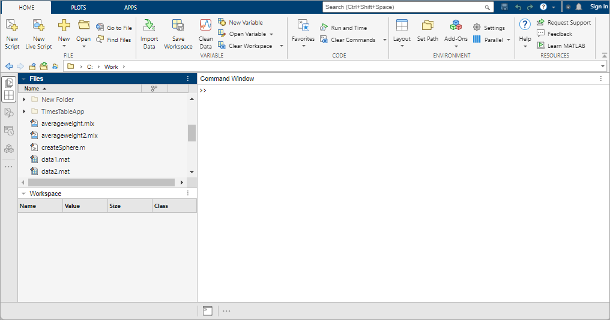## 桌面基础知识• 当前文件夹 - 访问您的文件。

• 命令行窗口 - 在命令行中输入命令（由提示符 (`>>`) 表示）。

• 工作区 - 浏览您创建或从文件导入的数据。

`a = 1`

MATLAB 将变量 `a` 添加到工作区，并在命令行窗口中显示结果。

```a = 1```

```b = 2 ```
```b = 2```
`c = a + b`
```c = 3```
`d = cos(a)`
```d = 0.5403```

`sin(a)`
```ans = 0.8415```

`e = a*b;`